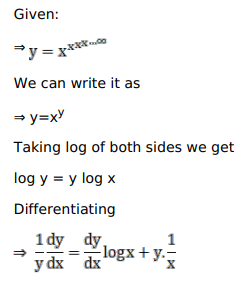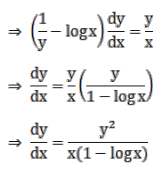# Solve this following

Question:

Mark $(\sqrt{)}$ against the correct answer in the following:

If $y=x^{x^{x \ldots x}}$ then $\frac{d y}{d x}=$ ?

A. $\frac{y}{x(1-\log x)}$

B. $\frac{y^{2}}{x(1-\log x)}$

C. $\frac{y}{x(1-y \log x)}$

D. none of these

Solution: